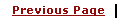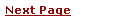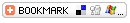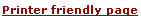C - Input and Output, I/OLearning C
C Function References
C Useful Resources
Selected Reading

Copyright © 2014 by tutorialspoint

 Home References About TP Advertising# C - Input and OutputAdvertisements

Input : In any programming language input means to feed some data into program. This can be given in the form of file or from command line. C programming language provides a set of built-in functions to read given input and feed it to the program as per requirement.

Output : In any programming language output means to display some data on screen, printer or in any file. C programming language provides a set of built-in functions to output required data.

Here we will discuss only one input function and one putput function just to understand the meaning of input and output. Rest of the functions are given into C - Built-in Functions

## printf() function

This is one of the most frequently used functions in C for output. ( we will discuss what is function in subsequent chapter. ).

Try following program to understand printf() function.

 ```#include main() { int dec = 5; char str[] = "abc"; char ch = 's'; float pi = 3.14; printf("%d %s %f %c\n", dec, str, pi, ch); } ```

The output of the above would be:

 ``` 5 abc 3.140000 c ```

Here %d is being used to print an integer, %s is being usedto print a string, %f is being used to print a float and %c is being used to print a character.

A complete syntax of printf() function is given in C - Built-in Functions

## scanf() function

This is the function which can be used to to read an input from the command line.

Try following program to understand scanf() function.

 ```#include main() { int x; int args; printf("Enter an integer: "); if (( args = scanf("%d", &x)) == 0) { printf("Error: not an integer\n"); } else { printf("Read in %d\n", x); } } ```

Here %d is being used to read an integer value and we are passing &x to store the vale read input. Here &indicates the address of variavle x.

This program will prompt you to enter a value. Whatever value you will enter at command prompt that will be output at the screen using printf() function. If you eneter a non-integer value then it will display an error message.

 ```Enter an integer: 20 Read in 20 ```

A complete set of input output functions is given in C - Built-in FunctionsAdvertisements

 Advertisements
Advertisements